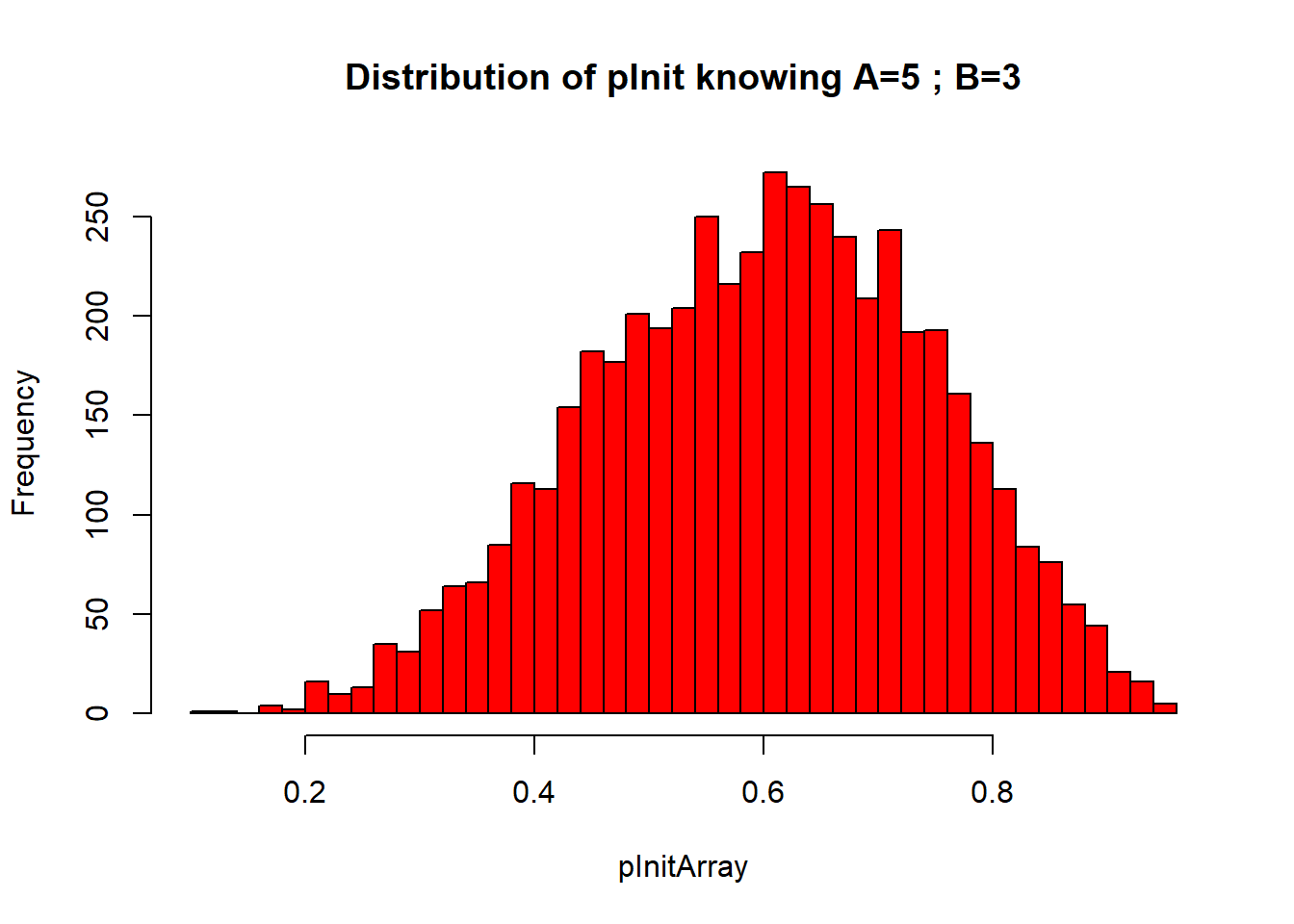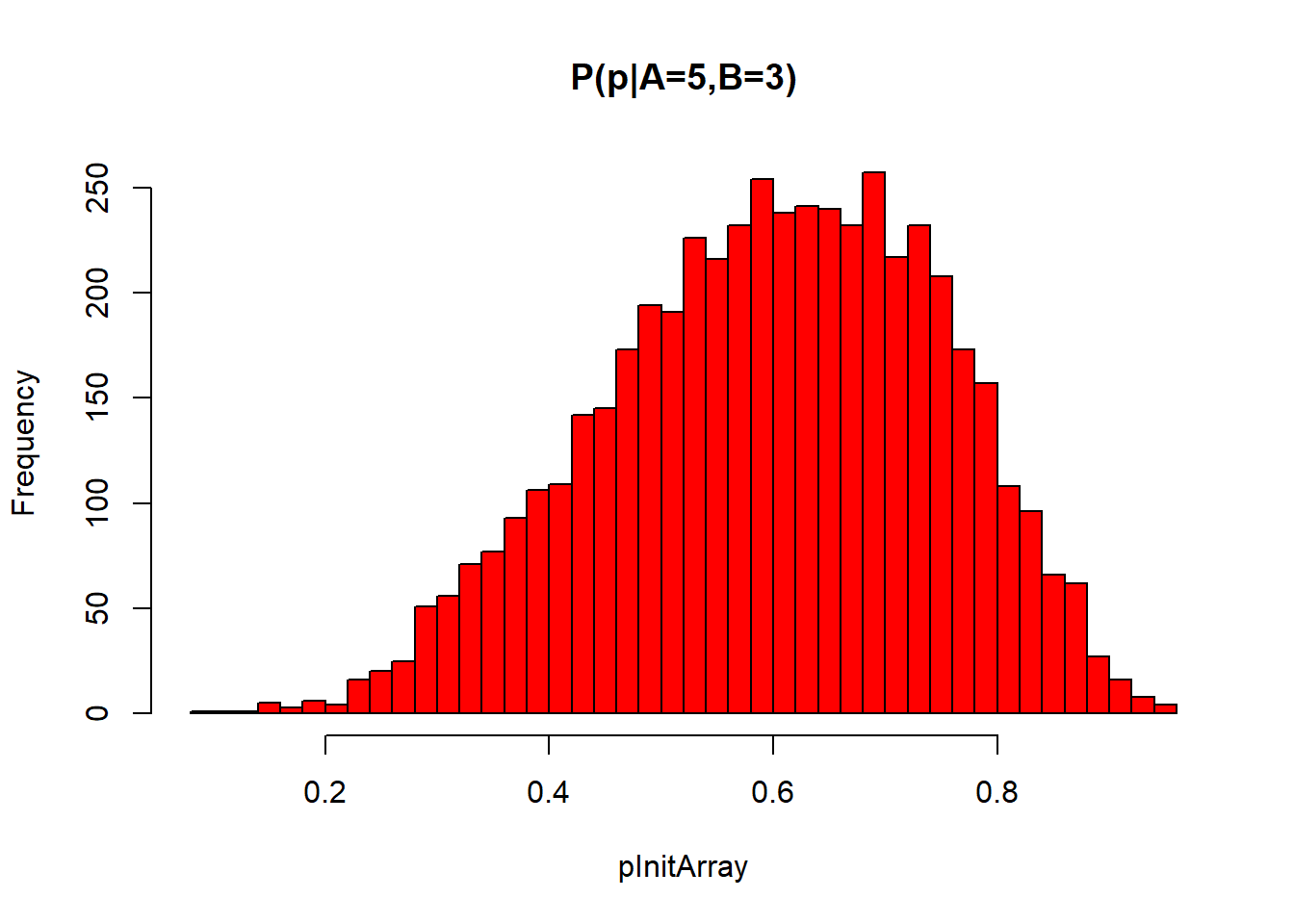### 1. Make the first toss to find the probability ($$p$$) that Alice wins in a single toss

• we cannot choose an arbitrary $$p$$ because we know that we obtained the intermediate observation $$A=5;B=3$$
• … so what we do is:
• choose a $$p$$ randomly between 0 and 1
• simulate 8 throws and count how often Alice won
• keep the $$p$$ if Alice won 5 times
logArray = rep(FALSE, 8)
while ( sum(logArray) != 5 ) {
pInit  = runif(1, min = 0, max = 1)   # choose some p from a uniform distribution on (0,1)
pNext8 = runif(8, min = 0, max = 1)   # throw 8 times, Alice wins every time the real number is below pInit
logArray = (pNext8 < pInit)           # how many times did Alice win? Loop stops if this is 5
}
sum(logArray) == 5   # this is TRUE now, i.e. we have a good pInit because Alice won 5 times with it
##  TRUE
cat(paste("The initial toss came up with p =", round(pInit,3) , "\n"))
## The initial toss came up with p = 0.285
• it is convenient to collect these commands in a function:
get_pInit <- function() {
logArray = rep(FALSE, 8)
while ( sum(logArray) != 5 ) {
pInit  = runif(1, min=0, max=1)
pNext8 = runif(8, min=0, max=1)
logArray = (pNext8 < pInit)
}
return(pInit)
}
• we can have a look at the empirical distribution of $$pInit$$
trials = 5000
pInitArray = numeric(trials )
for (i in 1:trials ) pInitArray[i] = get_pInit()
hist(pInitArray, col = "red", breaks = 40, main = "Distribution of pInit knowing A=5 ; B=3")  #  posterior distribution### 2. Play the game one time

• we start with the result already obtained: $$A=5;B=3$$
pInit = get_pInit()         # prior knowledge already included (via the distribution of pInit)
AlicePoints = 5             # current score
BobsPoints = 3
while ( (AlicePoints < 6) && (BobsPoints < 6)) {
nextThrow = runif(1, min = 0, max = 1)   # one toss
if ( nextThrow <= pInit) {AlicePoints = AlicePoints + 1} else {BobsPoints = BobsPoints + 1}
}
AliceWins = (AlicePoints == 6)
BobWins   = (BobsPoints == 6)
if(AliceWins) cat("Alice won.") else cat("Bob won.")
## Alice won.

### 3. Play the game many times

• now, play a lot of games and see how often both players win
• in every game, we choose a new initial $$p$$ by rolling the first ball (important!)
• we save the initial probabilities to check if the posterior distribution was correct
NumberAliceWins = 0
NumberBobWins = 0
numberGames = 5000
pInitArray = numeric(numberGames)

for (i in 1:numberGames) {
pInit = get_pInit()       # renew in each game!
pInitArray[i] = pInit     # save for histogram of posterior distribution
AlicePoints = 5           # current score
BobsPoints = 3

while ( (AlicePoints < 6) && (BobsPoints < 6)) {  # play this game until one participant wins
nextThrow = runif(1, min = 0, max = 1)
if ( nextThrow <= pInit) {AlicePoints = AlicePoints + 1} else {BobsPoints = BobsPoints + 1}
}
if(AlicePoints == 6) {NumberAliceWins = NumberAliceWins + 1} else {NumberBobWins = NumberBobWins + 1}
}
(NumberAliceWins + NumberBobWins) == numberGames  # This must be TRUE
##  TRUE

### 4. Results

• look at the posterior distribution generated above
hist(pInitArray, col="red", breaks=40, main="P(p|A=5,B=3)")• see what the odds is
odds = NumberAliceWins/NumberBobWins
cat(paste("The odds was", signif(odds, 3), "\n"))
## The odds was 9.92
• we cannot expect that the odds simulated here exactly agrees with the theoretical prediction
• neither will we obtain the same result if the algorithm is run repeated times
• however, in the vast majority of the cases, the result will be closer to the Bayesian prognosis
• the higher the number of games played, the bigger the probability that the result comes closer to the Bayesian prediction